# Convert Flat List to Table in Power BI; Pivot Without a Set Key Column Using Power Query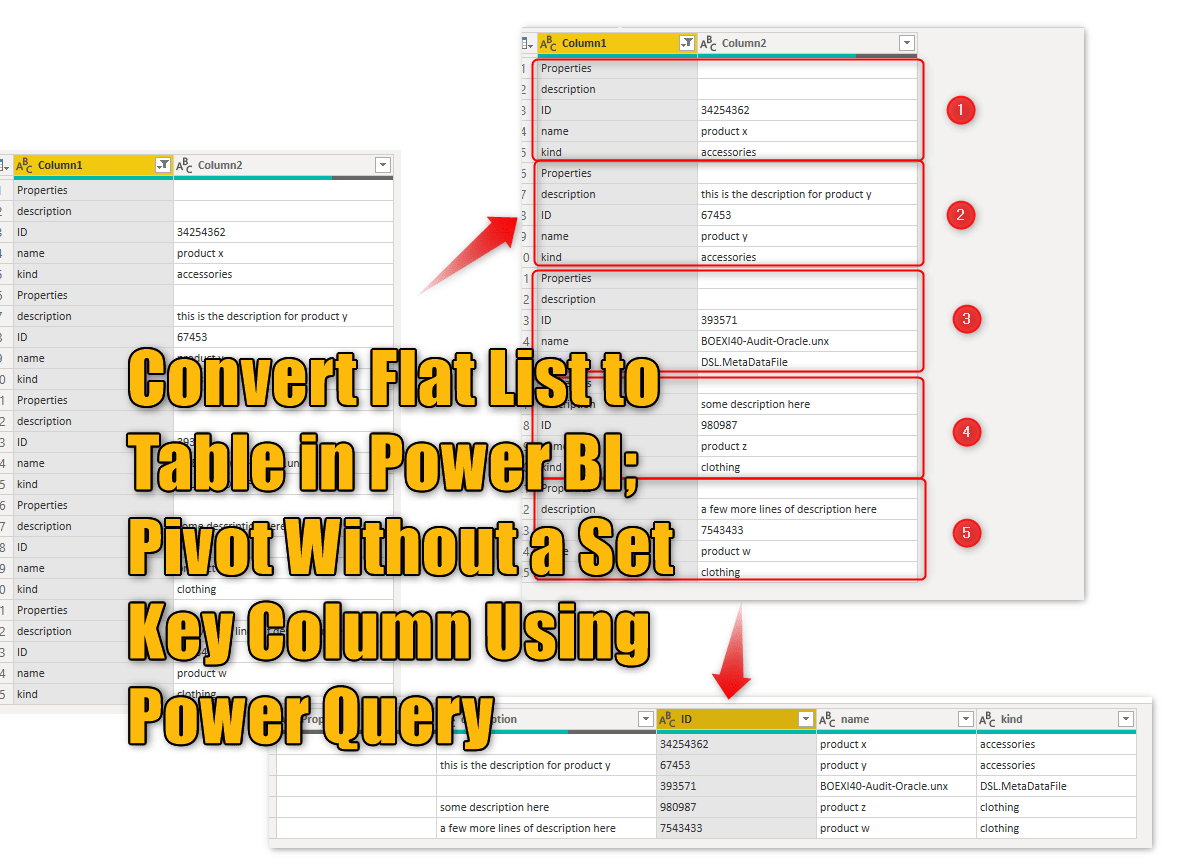The scenario I am explaining today is a very specific use case, However, I have seen examples similar to this happens very often. It happens that you get a text or Excel file with values written in a flat list structure, and that needs to be changed to a table format. Usually, this process can be done using PIVOT transformation in Power Query, however, sometimes, there is no key column to use for this operation, and the process is a bit more challenging. Let’s look at this example and see how this can be solved.

## The Scenario

Although, this sample scenario and this input data format are very specific. However, I have seen a pattern like this happens very often. So try to use the learnings of this pattern and apply it to similar cases.

I have a CSV file with the data like below: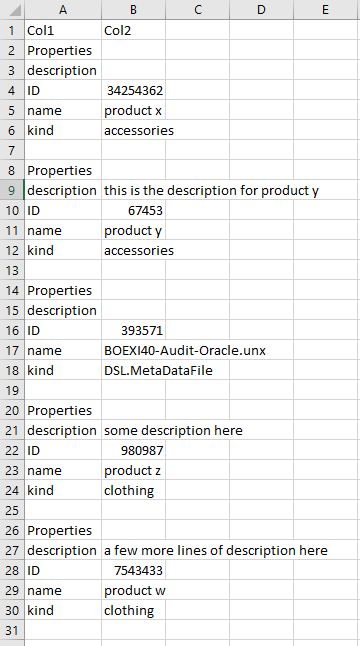The data above needs to be re-shaped like below to become useful for analysis: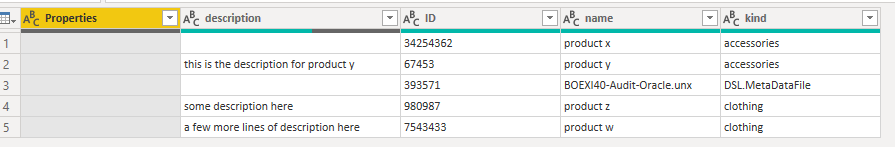## The Challenge

Often when you have data in rows and you want to convert it to columns, PIVOT is a useful transformation. Pivot will change rows to columns considering a column to be the pivot key, and another column to have values, and one or more other columns to produce one value per key/value pair. the other column(s) are kind of necessary to get useful output, otherwise, PIVOT will return an error. To show you how pivot would react in the existing dataset, I’ll do some steps to clean up the data first.

### Cleaned Dataset

I removed the first row, as it didn’t have any useful information, and removed empty values in the first column. here is my dataset cleaned as below so far;Pivoting this dataset will give you an output like this (if you use the Don’t Aggregate advanced option)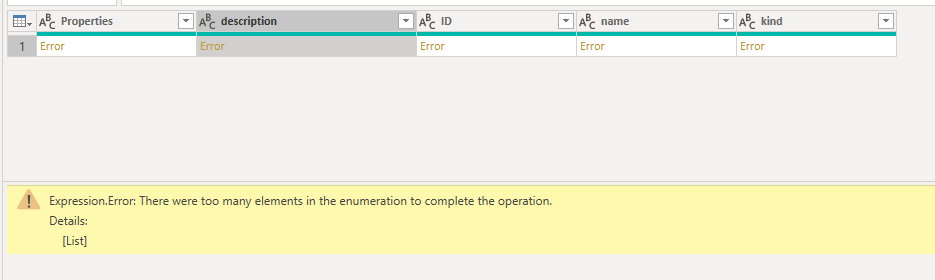and if you use Pivot with an aggregation, then all you get is the count of rows, or the maximum item, or minimum, etc. you won’t get the details of each row. so here is the challenge. Now let’s fix it.

## The Solution

If we had a column that had unique values per each combination of rows in the flat list, then the Pivot transformation won’t fail. we need to somehow, change the dataset to add that column. this column should provide an index for each set of groups as below;We can use the ID column in the dataset, however, there is an easier way to do it. Considering that Properties is always the label of the first field in each set, we can start numbering the records using the Properties. However, you cannot add an index column conditionally. so here is how we can do it:

You can simply add an index column (doesn’t matter start from zero or one or any other custom options);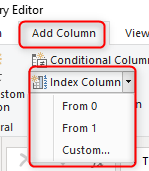here is the index added so far;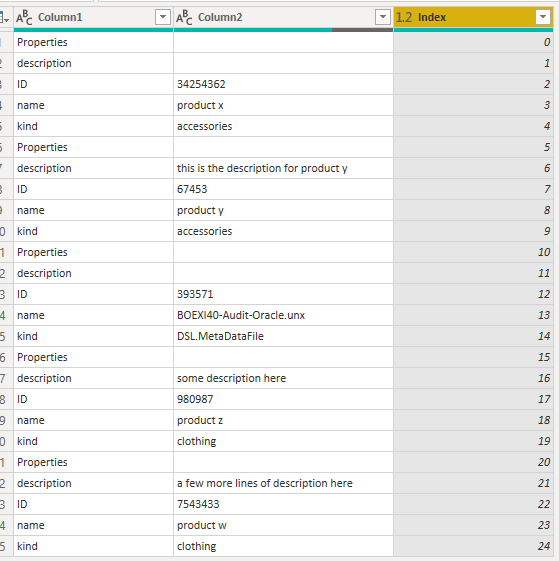if we always know that each set has five fields, we could simply use a divide or mod from the index, but let’s say that is not the case.

The Set header is the values “Properties” in Column1, because with the appearance of that a new set starts. you can add a conditional column for this check and return the index only if this condition is met.this leads the result of index column only for the set headers:### Fill Down; Repeating set header value for the entire set

Now that you have a unique value per set headers, you can use a Fill Down transformation to fill all null values with that value.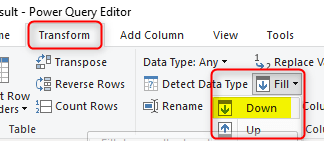As a result, now we have the set key column for each row;We don’t need the Index column anymore, and can call this column Key;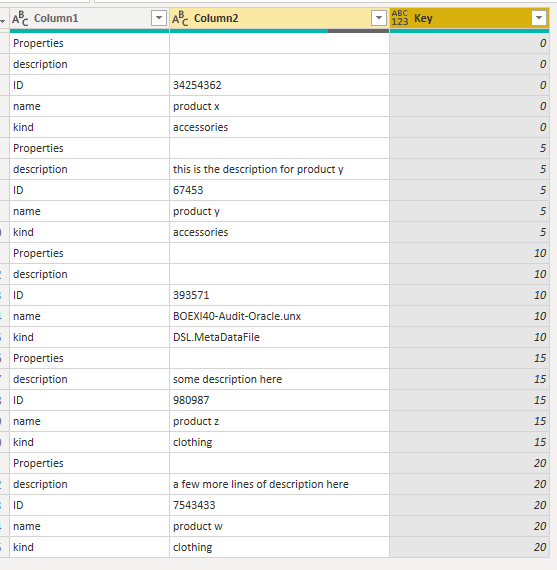### PIVOT: Rows to Columns

Now that you did all the preparation and added the set key column, you can simply use the Pivot transformation;The pivot key column is the Column1 that includes field names, the value column is the Column2 that includes values. Set the aggregation function as Don’t Aggregate. You might wonder, then where the Key column (the column that we created in the previous steps) is used? the answer is: That column is used behind the scene for the pivot function to stop aggregating all rows to each other.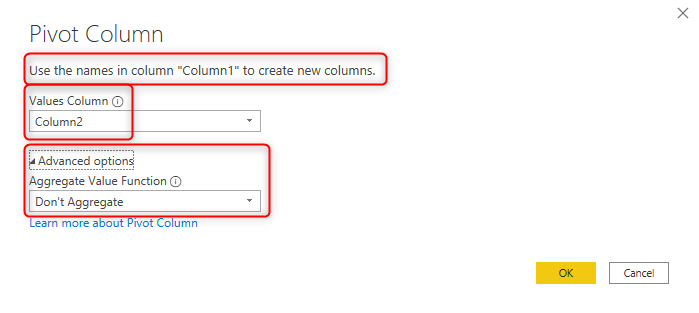And here is the result now;The Key column is not needed anymore. you can remove it to have the final dataset;## Summary

As I mentioned, the approach explained in this scenario is unique, and you might not have exactly this kind of data to work with. However, often, you might find similar patterns of data where you need to pivot the data, but there is no key column to stop the aggregation error. The method used here is one of the ways to assign a unique key for each set of fields (which will become a data row in the desired output) and uses that for the pivot transformation.